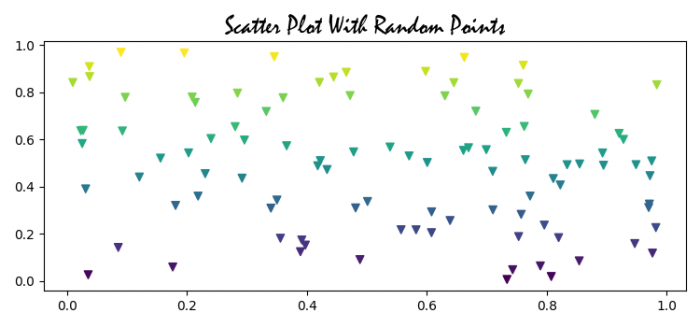# How to embed fonts in PDFs produced by Matplotlib

To embed fonts in PDFs produced by Matplotlib, we can use rc.Params['pdf.fonttype']=42.

## Steps

• Set the figure size and adjust the padding between and around the subplots.
• Create a new figure or activate an existing figure, using figure() method.
• Create x and y data points using numpy.
• Plot x and y data points using scatter() method.
• Set the title of the plot.
• Save the figure in pdf format.

## Example

import numpy as np
from matplotlib import pyplot as plt, font_manager as fm

plt.rcParams["figure.figsize"] = [7.50, 3.50]
plt.rcParams["figure.autolayout"] = True
plt.rcParams['pdf.fonttype'] = 42

fig, ax = plt.subplots()
x = np.random.rand(100)
y = np.random.rand(100)

ax.scatter(x, y, c=y, marker="v")

fprop = fm.FontProperties(fname='C:\Windows\Fonts\MISTRAL.TTF')

ax.set_title('Scatter Plot With Random Points',
fontproperties=fprop, size=20, fontweight="bold")

plt.savefig("demo.pdf")

## Output

When we execute the code, it will save the following plot in the project directory as "demo.pdf".Observe the font style of the title. We have set the title in Mistral font

Updated on: 16-Jun-2021

688 Views